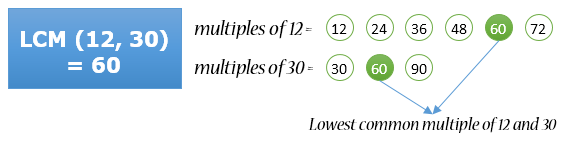# Functions Exercises

## C program to find LCM of two numbers using recursion

Write a C program to find LCM of two numbers using recursion. How to find LCM of two numbers in C programming using recursion. Logic to find LCM of two numbers using recursion.

#### Required knowledge

Basic C programming, If else,for loop,Functions, Recursion

#### Logic to find LCM of two numbers using recursionFinding LCM using iterative method involves three basic steps:

1. Initialize multiple variable with the maximum value among two given numbers.
2. Check whether multiple clearly divides both number or not. If it does, then end the process and return multiple as LCM.
3. If multiple doesn't divides both given numbers then increment multiple by the max values among both given numbers.
Repeat steps 2 to 3 till you find LCM. To convert the above iterative approach of finding LCM into recursive we will use step 2 as our base condition.

#### Program to find LCM using recursion

```
/**
* C program to find LCM of two numbers using recursion
*/

#include <stdio.h>

/* Function declaration */
int lcm(int a, int b);

int main()
{
int num1, num2, LCM;

/* Input two numbers from user */
printf("Enter any two numbers to find lcm: ");
scanf("%d%d", &num1, &num2);

/*
* Ensures that first parameter of LCM function
* is always less than second
*/
if(num1 > num2)
LCM = lcm(num2, num1);
else
LCM = lcm(num1, num2);

printf("LCM of %d and %d = %d", num1, num2, LCM);

return 0;
}

/**
* Recursive function to find lcm of two numbers 'a' and 'b'.
* Here 'a' needs to be always less than 'b'.
*/
int lcm(int a, int b)
{
static int multiple = 0;

/* Increments multiple by adding max value to it */
multiple += b;

/*
* Base condition of recursion
* If found a common multiple then return the multiple.
*/
if((multiple % a == 0) && (multiple % b == 0))
{
return multiple;
}
else
{
return lcm(a, b);
}
}

```

Note: Since we don't want the variable multiple to re-initialize again and again by recursive function calls, therefore I have declared it as static i.e. static int multiple = 0;.

Also you can remove the other condition as it is not necessary. Means you can simply write the if statement as if(multiple % a == 0). Since the variable multiple is always a multiple of b.

#### Output

Enter any two numbers to find lcm: 12

30

LCM of 12 and 30 = 60